# Control: The "Uncle Fester" of the Data Science Family (part 2--Optimization)"Darling, this must be really bothering you. The last time you shook Uncle Fester like that you were trying to get the termites out of his sinuses."

Firstly, let's review the outline for this series:

• Part 1: Deals with a general taxonomies of data science and simulation science, and how the two complement one another. It also identifies control as a key component of both data science and simulation science.
• Part 2 (this part): Deals with a taxonomy of optimization. Identifies optimization and data fitting (e.g. regression analysis) as the same problem.
• Part 3: Moves into the theory of control.
• Part 4 (pending): Deals with difficult issues in control in the real world; things like selecting the best controls in the absence of important information, or in the presence of deceptions in the operating environment.

So part 1 of this series provided a taxonomy of data science that aligns well with the Data/Information/Knowledge/Wisdom Pyramid, dividing data science into three complementary components:

1. Data Fusion: Transforms observations of the world into estimates of variables of enterprise interest.
2. Analytics: Applies business rules to variables of interest giving answers to enterprise questions.
3. Control: Uses the values of enterprise variables and answers to enterprise questions to inform decisions and actions to further enterprise goals.

For that discussion, we treated control and optimization as the same thing and used the word "control" as shorthand for both. But we promised, at the end, to illuminate the distinctions between optimization and control, starting with a more structured discussion of optimization. That is our subject here. By some miracle of Nature, or cosmic mathematical coincidence, there are three extremely important problems that end up being identical:

1. Finding the best fit to a set of data (regression),

2. Finding the places where the a system or function takes a certain value (root finding),

3. Finding the minimum or maximum value of a system or function (optimization).

The regression problem is obviously crucial to data science. It’s the bread-and-butter of data fusion. Regression is how the enterprise gets its situational awareness. Root finding comes up in analytics where we answer enterprise questions. Those questions often take the form of "when does this thing equal that thing?" As a trivial example, a phase space in which a system operates is often partitioned into stable and unstable regimes. One of the most important enterprise questions is whether we are operating in a stable regime or an unstable one. Root finding is needed to find the boundaries of those regimes. So root finding is key to situational understanding. Optimization is key to data science because it is how the enterprise transforms situational understanding into effective decisions.

If you can solve any of these problems, you can solve all of them. They are the same. Unfortunately, that problem is tricky. Typically, there is some kind of a cheat. For instance, in regression problems we usually restrict the model we fit to. Linear fits are easy. And lots of things are linear, but the most interesting things are not "steady as she goes!" Part of the data scientist's job is to know which cheats are being used and what the impact of those cheats are. Many businesses have ridden bad forecasts into bankruptcy not even realizing that they were depending on a linear model to describe a nonlinear environment. This exemplifies a common pattern of error worth mentioning. We often have the choice to either solve the right problem approximately or the wrong problem exactly. Usually getting the exact answer to the wrong problem started out being a way to get an approximate answer to the right problem, but somewhere along the line we lost our way and forgot which problem we were actually trying to solve.

Since regression, root finding, and optimization are the same, the optimization problem is a good one to use to describe the twists in the road that affects all of these problems. The optimization problem is to find the parameters that give the best value of a function known as the “objective function”. Mathematicians tend to treat the optimal value of the objective as a minimum while engineers treat it as a maximum. But it doesn’t matter. The math works out the same either way. So for purposes of this discussion, let’s say that the problem is to minimize the value of the objective function, which we will call f(x). And we call the value of the optimal parameter x*.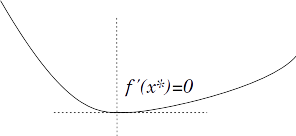Elementary calculus and the figure above tell us that the minimum, x* will be at a place where the first derivative vanishes. I.e.

x* is chosen such that  f’(x*)=0.

That is, the optimal point occurs where the curve read left-to-right stops going down. By the way, this is our old friend the root finding problem, which probably isn't a surprise since we said that problem was somehow equivalent to optimization. Setting  f'(x)=0  is a good start, and it goes a long way, but there are lots of special cases and in practice all of the interesting problems involve some and usually several of these conditions. Like:

i) No minima exists:

What if f(x) just keeps diminishing (thus f’(x) is never 0) like in a straight line? Indeed, some objective functions have no minima, We need to recognize these cases.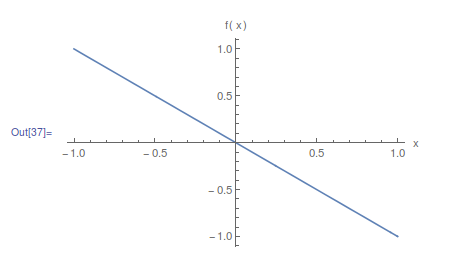ii) Asymptotic convergence to a minima:

What if the value of f(x) continues to diminish for all time, like with the line, except in some limiting “asymptotic” way, like where f(x)=e-x. We need to be able to tell when these conditions come about. Energy functions exhibit this kind of behavior. In fact the universe itself may be following this kind of curve.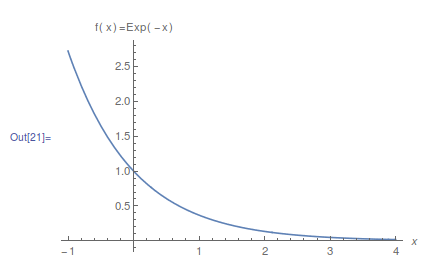iii) Corners--f’(x) is undefined at the minimum:

Just because f’(x) is never 0 doesn’t mean that there is no minimum. If the function has corners, f’(x) is undefined at a corner, and corners are often (but not necessarily) associated with some kind of minimum or maximum. That’s what happens with the absolute value function: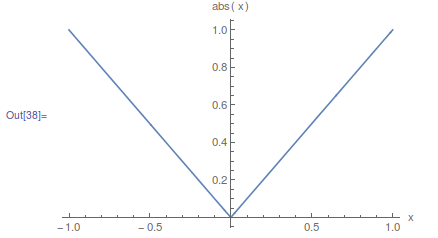iv) Inflection Points:

Not every point where f’(x)=0 is a minima or maxima. What if f’(x)=0 because the function temporarily levels off, but then starts going down again before it goes up? The simple function  f(x)=-x3  is one of these "washboard" curves. We need some additional conditions and higher order derivatives to recognize and study these cases.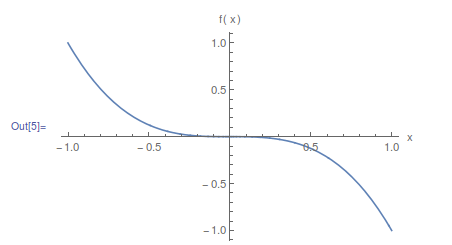v)  Multiple extrema:

What if there are multiple places where f’(x)=0, like if f(x) is a quartic? We need to know how to enumerate those points, and we need to know when we have all of them or at least the right ones. These kinds of functions arise in beam stresses and other “boundary value problems”. Quartics are one of the simplest cases of multiple extrema (or at least of multiple minima), and it is true that quartics can be solved analytically, like the quadratic formula except with lots more terms. In fact we only need to solve a cubic to get the extrema of a quartic. But in another devilish turn of mathematical fate, just a little more complexity ruins all of those hopes. There is no general analytic solution to the quintic (fifth order polynomial), let alone transcendental functions. In practice, we use higher-order polynomials to approximate more complex functions and particularly to approximate data series acquired through observations. They're really good functions, but you're still left to numerical methods to find their roots.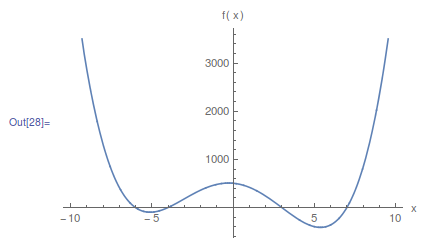vi) Vector-valued functions:

What if x isn’t a single scalar value but is instead a vector, as in f(x1,x2)=x12+x22? Suddenly we have more derivatives (now partial derivatives) and things get messier even for simple cases.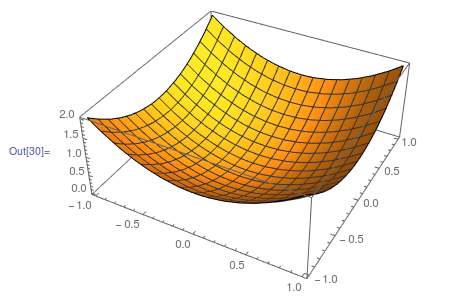If x is a vector, then a point can look like a minimum in one dimension and a maximum in another, as in f(x1,x2)=x12-x22. Once again, we need more conditions and higher-order derivatives to deal with “saddle points”: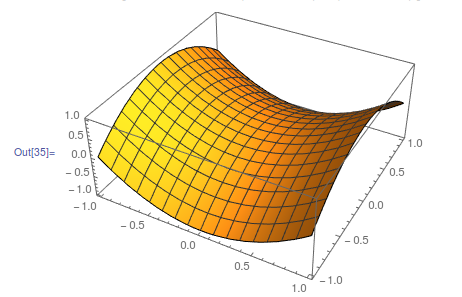viii) Combinatorial optimization:

What if x is only meaningful at integer values and so the derivative f’(x) simply doesn’t exist anywhere? Say, for example, we are packing for a trip and we want to know the best number of socks and shoes and shirts to take with us and still fit everything in one bag.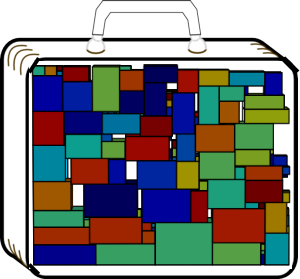ix) Variational problems:

What if x isn’t a number at all, and is instead a function? Like if we are trying to find the shape of a skateboard ramp that takes you from point A to point B in the minimum amount of time. That particular problem is called a “brachistochrone” problem, Greek for “least time”. It can be solved. The classic tool is known as Calculus of Variations. Nature seems to have a little computer for solving these problems on her own. Consider a wire ring that has been twisted a bit. Now we span that ring with a soap film. Nature determines the shape the soap film takes. That shape will minimize the overall surface tension. So it's a well-formed optimization problem, and the calculus of variations can be used to compute that shape: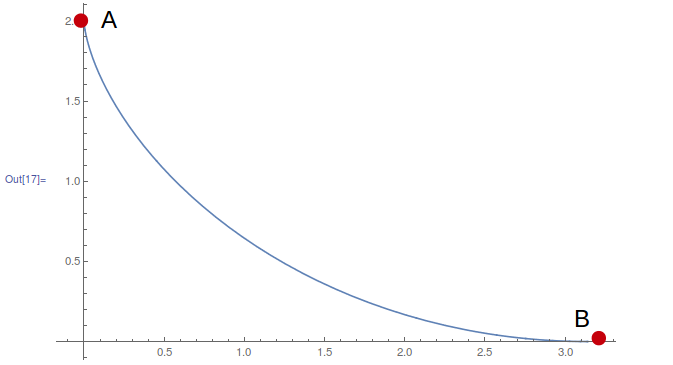x) Constrained optimization:

What if there are restrictions on the parameters? Say we are selling “Grade A” almonds and we want make the mix at minimum cost, but the USDA says that you can only sell almonds as “Grade A” if there are fewer than 4% splits and less than 0.5% rot, and that 80% of them need to be larger than 20mm. Constraints can often be dealt with, but they add a lot of complexity. The constraints, taken together, create a “feasible region” where all constraints are satisfied, like in the figure below where all of the constraints are linear. In the interesting cases, the optimum ends up being on the boundary of the feasible region rather than in its interior. By yet another cosmic mathematical coincidence, this can be handled by changing the objective function rather than changing the way we search (Karush-Kuhn-Tucker Theorem). Unfortunately, those changes to the objective function can be daunting.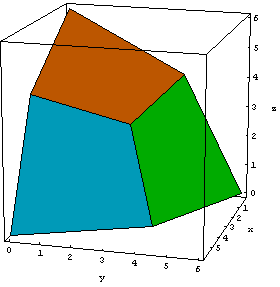xi) Stochastic optimization:

What if the data is fraught with uncertainty? Like if you want your stock portfolio to give a certain return with minimum overall risk (image from the open source PyFolio project).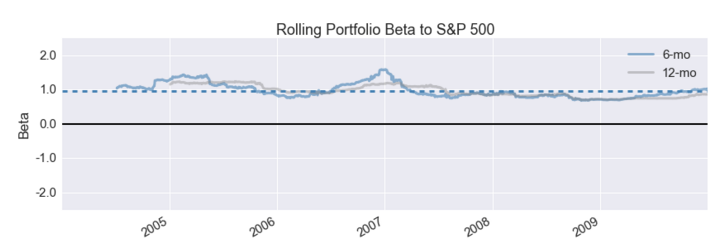xii) Sensitivity:

What if the absolute minimum depends on some touchy parameters while there are other choices that are nearly as good but are much more stable: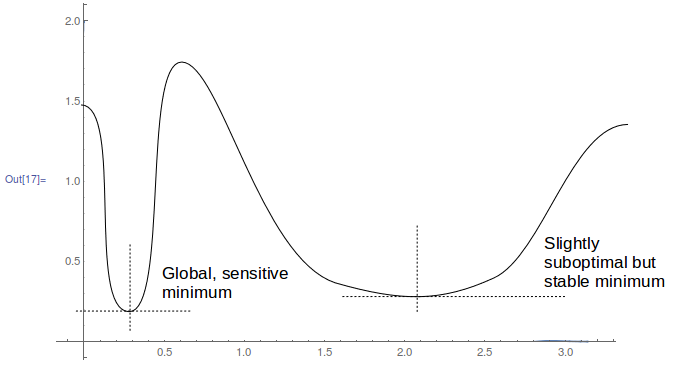So there is a lot more than elementary calculus going on here. But we can make a couple of claims that are easy to understand and quite powerful.

1. The “No Free Lunch” Theorem: The No Free Lunch Theorem says that when averaged over all optimization problems, no approach can do better than randomly searching for the best answer. So you need to pick your approach based on the specific problem you are trying to solve. This requires some practice and knowledge, and maybe some trial-and-error.

2. Global Optimization is Hard: Finding the global (overall best) optimum is an extremely hard problem; much harder than finding a local (better than anything around it) optimum. So finding a local optimum isn’t usually cause to declare victory. In fact it’s usually a nuisance because we get “stuck” in a local optimum while searching for the global one. Imagine, for a moment, that you’re standing at a canyon rim and want to find its bottom. If there were no local minima, you could simply drop a marble and let it roll down the canyon wall. When it finally came to rest it would be at the bottom. This is not so different from the optimization method known as “gradient descent”. But if there are crags and gullys the marble won’t make it very far. From the marble’s perspective under the guidance of gravity, a divot in the sand is indistinguishable from canyon’s bottom, because gravity only knows how to find a local minima.

3. Combinatorial Optimization is also Hard: Another of our cosmic mathematical coincidences involves problems like picking the clothes to pack and packing them in a suitcase, or planning an optimal route or schedule. These fall--with curious regularity--into the complexity class called NP-Complete which contains computing problems that can technically be solved by enumerating all possibilities and picking the best, but cannot be solved practically because the time required to enumerate those possibilities, even for small problems, exceeds the lifetime of the universe, and no other faster ways to solve those problems is known. In fact, it is highly likely that there are no faster ways to solve these problems exactly, so no matter how smart we are or how long we try, we will never find a faster way because there is none. There is an important misconception here that deserves a response. When Peter Shor revealed his quantum algorithm for factoring integers, the popular and some technical media reported that this proved that quantum computers could solve the NP-Complete problems trivially. That argument relied on an assumption--one that Shor never made--that factoring integers was as hard as planning routes or packing suitcases. While that isn’t the case (factoring integers is easier), the hype has stuck and popular opinion on the subject has been slow to change. What is true is that unless we learn that a lot of accepted mathematics is wrong, quantum computers will never solve NP-Complete problems in trivial amounts of time as reported in the popular press.

So optimization is hard to do. In fact, the “skateboard ramp” example above was the equivalent of an XPrize problem in the late 17th century. Isaac Newton and Johann Bernoulli solved the problem in 1697, and that work inspired much of what we now call calculus. Much of 20th century mathematics was devoted to more general methods of optimization, but there are no magic bullets. The current state-of-the-art typically applies heuristics--rules of thumb--that, in the right hands, can be used to get very good approximations to optimal solutions. Heuristics are particularly useful for combinatorial problems like routing and scheduling where no calculus-based derivatives exist. We usually exploit derivatives from calculus wherever possible because they are the best indicator of a minimum. If we have derivatives, we use them to describe the shape of the function. The two most common uses are “line search” and “trust region search”.

• Line Search: In a line search, we assume that the function describing the derivatives is straight, at least near some sample point or “guess”, and then we follow the line, searching for the place  where it is zero, and use that as a next guess for where the derivative is zero, and go again until we get close enough. The closer the derivative resembles a line, the better this works. But if the initial guess isn’t good enough, the process can diverge and never find a solution, or perhaps worse (if you rely on it) find the wrong solution.
• Trust Region Search: In a trust region search, instead of following a line to the next guess, we model the region near the guess as a surface, like a bowl. Then we find the bottom of the bowl and use that to inform the next guess.

It’s easy to see how either of these methods could get stuck in a local minima, and that’s a big issue. But even if there are no local (i.e. non-global) minima, things can be difficult. Consider how a line search would work if you were trying to find the bottom of this helical coil. The Helical Coil problem, is not to find the lowest point on the coil (that one's hard enough), it's to find the lowest point of the inside of the tube that makes up the coil. From the perspective of a tiny camera inside the tube, the world looks round and almost straight and almost flat.This is a problem where dropping the marble in the tube would actually work, so by some useful measure, this is an easy problem: the derivatives are all defined and there are no local minima. But in line search, you would find the bottom of the tube (not the coil), and nudge your way along the tube finding the bottom of the tube again and again, a little further ahead perhaps millions of times before you got to the bottom of the coil. Some variations of line search are better than others at finding their way down the coil, but the issue is that the line search doesn’t know it’s a coil. All it sees is the tube. The same thing holds for trust region search.

If there are no derivatives, like in combinatorial optimization, things get harder. Most combinatorial optimization uses a variation on the trust region method where we define a family of possible solutions that are in some sense “near” our initial guess, and we see if any of these are better than the guess. If so, we update the guess. But there is tremendous cleverness in how we define what “near” means. One way to do this is to represent any solution as a “chromosome”. We maintain a population of “fit” individuals or chromosomes (meaning that they give low values for the objective function) and then we select pairs of fit chromosomes to “recombine” in a process of Darwinian evolution, giving the most fit offspring a higher likelihood of producing their own offspring. The trust region is the line between to the two "parents" in chromosome space, and contains the set of chromosomes that could be created by combining the two parents. These “genetic algorithms” sound hopelessly slow, but while they are tedious they do work for some problems, so long as the evolutionary process is designed and implemented by skilled practitioners who understand the nuances of the specific problem. A similar heuristic (simulated annealing) mimics the energy minimization process that occurs when a glass or metal is cooled very slowly. The slower the cooling schedule, the better the result. Unfortunately, this may take a very very long time to get good results. But like the genetic algorithm, it does work, for some problems, when carefully designed.

All of this means that our “in the right hands” qualifier is critical. It’s good that optimization software exists, and it’s good that so much of this software is available through open source. But one reason so much is in open source is that having the software installed--even if it’s the right software--doesn’t get you much closer to the answer. The quality of the solution still depends on using it with informed judgement.

The optimization examples we’ve given are “one shot” problems: pack a bag, mix a batch of nuts, make a skateboard ramp, design a portfolio, etc. But many real world problems require multiple steps or the continuous application of force, and we want to solve these optimally, too. How much jet fuel should we be burning at any point in time to complete a transoceanic flight with minimum fuel cost? How much force should be applied to the brakes moment-to-moment to stop in the minimum distance? What is our strategy for winning at chess, or battleship, or building market share over the next three years? These are control questions, and they will be addressed in the next post, on control.

Views: 1359

Comment

Join Data Science Central